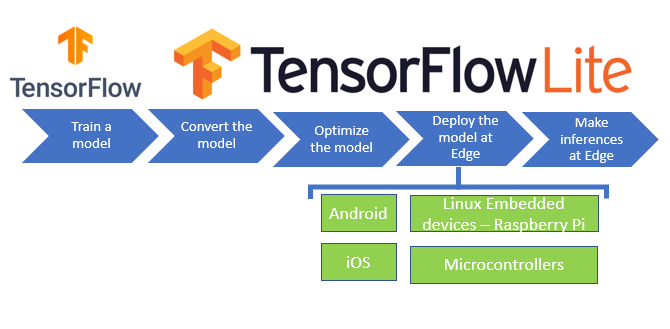# Deploy Tensorflow model 4x faster

Hello, For a couple of weeks I was trying to deploy the TensorFlow model (hand digit recognition) with edge devices. Within this post, we are going to discuss how we can deploy the TensorFlow model on edge devices like jetsonNano or any edge device.Workflow for the deployment of TensorFlow model

I would advise you that always consider model optimization during your application development process. This document outlines some best practices for optimizing Tensorflow models for deployment on edge hardware

# Why we need optimization?

Optimization brings your model Smaller in Size, And at the same time, it should have low computation to run inference, which will lead me to low Latency.

We have different types of optimization techniques in TensorFlow.

# Types of optimization

TensorFlow currently supports optimization via

quantization

pruning

clustering.

# Quantization

Quantization works by reducing the precision of numbers used to represent the model’s parameter, which is by default 32bit floating points numbers.

# Dynamic range quantization

This is the simplest form of post-training quantization statically quantizes only the weights from floating-point to integer, which has 8-bits of precision.

here, I am going to show you a short code snippet that can give you an idea of how I have used TensorFlow optimization.

`# import tensorflowimport tensorflow as tfimport tensorflow_model_optimization as tfmot..model.fit (train_image,train_labes,epochs=1,validation_data=(test_images,test_labels))######### initialize quatization aware model ###################### q_aware stands for for quantization aware.q_aware_model = quantize_model(model)########## `quantize_model` requires a recompile.################q_aware_model.compile(optimizer='adam',         loss=tf.keras.losses.SparseCategoricalCrossentropy(from_logits=True),              metrics=['accuracy'])q_aware_model.summary()#################### Train Model ###########################train_images_subset = train_images[0:1000] # out of 60000train_labels_subset = train_labels[0:1000]q_aware_model.fit(train_images_subset, train_labels_subset,                  batch_size=500, epochs=1, validation_split=0.1)###################### evaluate the model#################_, baseline_model_accuracy = model.evaluate(    test_images, test_labels, verbose=0)_, q_aware_model_accuracy = q_aware_model.evaluate(   test_images, test_labels, verbose=0)print('Baseline test accuracy:', baseline_model_accuracy)print('Quant test accuracy:', q_aware_model_accuracy)################### save this model##################### _, keras_model_file = tempfile.mkstemp('.h5')q_aware_model_accuracy.save(keras_model_file)################### Load Model########################## `quantize_scope` is needed for deserializing HDF5 models.##with tfmot.quantization.keras.quantize_scope():  loaded_model = tf.keras.models.load_model(keras_model_file)loaded_model.summary()`

# Create a quantized model for the TFLite backend

`converter = tf.lite.TFLiteConverter.from_keras_model(q_aware_model)converter.optimizations = [tf.lite.Optimize.DEFAULT]quantized_tflite_model = converter.convert()with open(quant_file, 'wb') as f:  f.write(quantized_tflite_model)`

Now we can use these trained tflite models in my desire place on android or edge.